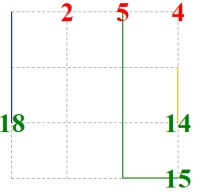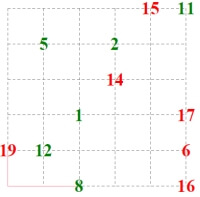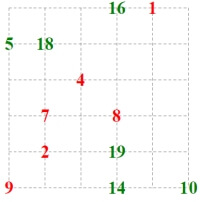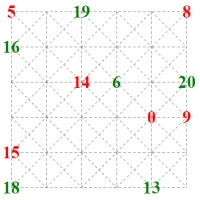edHelper.com Math Worksheets Make Puzzles Critical Thinking Kissing Activity - Number, Letter, and Picture Kissing Activity Printable Lesson Plans Number Kissing Activity - Greater and Less ThanWorksheets, Lessons, and Printables

Number Kissing Activity - Greater and Less Than

Number Kissing (one line given and partial lines)Number Kissing - 4 by 4 - one line given and partial answers Number Kissing - 5 by 5 - one line given and partial answers Number Kissing - 6 by 6 - one line given and partial answers Number Kissing - 7 by 7 - one line given and partial answers Number Kissing - 10 by 10 - one line given and partial answers

Number Kissing (one line given)Number Kissing - 4 by 4 - one line given Number Kissing - 5 by 5 - one line given Number Kissing - 6 by 6 - one line given Number Kissing - 7 by 7 - one line given Number Kissing - 10 by 10 - one line given

Number KissingNumber Kissing - 4 by 4 Number Kissing - 5 by 5 Number Kissing - 6 by 6 Number Kissing - 7 by 7 Number Kissing - 10 by 10

Number Kissing (with one diagonal page)Number Kissing - 4 by 4 - one diagonal page Number Kissing - 5 by 5 - one diagonal page Number Kissing - 6 by 6 - one diagonal page Number Kissing - 7 by 7 - one diagonal page

Kissing Activity

Have a suggestion or would like to leave feedback?
Leave your suggestions or comments about edHelper!